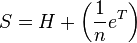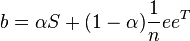A Google matrix is a particular stochastic matrix which is used by Google‘s PageRank algorithm. The matrix represents a graph with edges representing links between pages.

The rank of each page can be generated iteratively from the Google matrix using the power method. However, in order for the power method to converge, the matrix must be stochastic, irreducible and aperiodic.

### H Matrix

In order to generate the Google matrix, we must first generate a matrix, H representing the relations between pages.

Assuming there are n pages, we can fill out H by doing the following:

1. Fill in each entry (i,j) with a 1 if node i has a link to node j, and 0 otherwise.
2. Divide each row by ki where ki is the total number of links to other pages from node i.

H is usually not stochastic, irreducible, or aperiodic, making it unsuitable for the PageRank algorithm.

### G Matrix

Given H, we can generate G by making H stochastic, irreducible, and aperiodic.

We can first generate the stochastic matrix S from H by adding an edge from every sink state to every other node. In the case where there is only one sink state, S written as:where a is the number of nodes.

Then, by creating a relation between nodes without a relation with a factor of α, the matrix will become irreducible. By making H irreducible, we are also making it aperiodic.

The final Google matrix can be computed as:If combined with the H computed above and with the assumption of a single sink node, the Google matrix can be computed as:Although G is a dense matrix, it is computable using H which is a sparse matrix.

For the actual matrix, Google uses an α around 0.85.956.